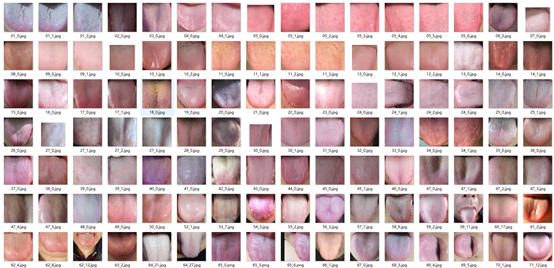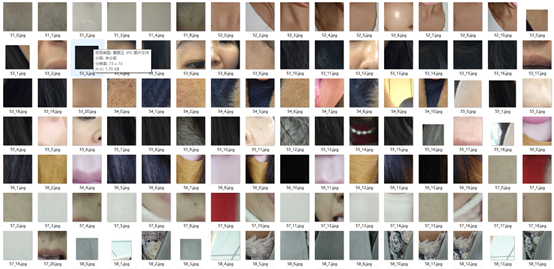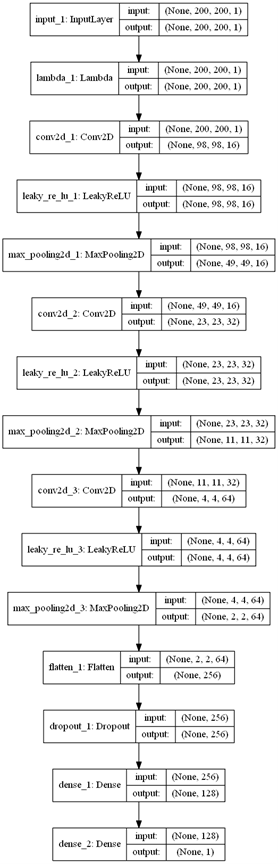# opencv舌头监测模型+keras神经网络（LeNet）

## 收集整理数据集## 构建卷积神经网络并训练import cv2
import os
import numpy as np
import tensorflow as tf

import keras.backend as K
from keras.datasets import mnist
from keras.layers import *
from keras.models import *
from keras.optimizers import *
from keras.initializers import *
from keras.callbacks import *
from keras.utils.vis_utils import plot_model#显示层级图
from tqdm import tqdm

"""
获取灰度值图片
:param path:
:return:
"""
img = cv2.cvtColor(img, cv2.COLOR_BGR2GRAY)
img = cv2.resize(img, (shape, shape))
return np.reshape(img, shape)

"""
加载数据集
:param dir:
:return:
"""
imgs = []
for fn in os.listdir(dir):
if fn.endswith('jpg'):
return np.array(imgs)#转换为numpy矩阵

def net():
"""卷及网络模型"""
inputs = Input(shape=(200, 200, 1))
model = Lambda(lambda x: (x - 127.5) / 127.5)(inputs)#将像素值变为（-1，-1）----灰度值是从0-255
#卷积层--16个特征图，关机过滤器（5*5）,步长2*2，特征图大小：（200-5+2）/2=98
model = Conv2D(16, 5, strides=(2, 2))(model)
# LeakyReLU仍然会有非零输出值，从而获得一个小梯度，避免ReLU可能出现的神经元“死亡”现象。即，f(x)=alpha * x for x < 0, f(x) = x for x>=0

#  sigmoid和tanh在x趋于无穷的两侧，都出现导数为0的现象，成为软饱和激活函数。也就是造成梯度消失的情况，从而无法更新网络状态。
# relu的主要特点就是：单侧抑制，相对宽阔的兴奋边界，稀疏激活性。稀疏激活性，是指使得部分神经元输出为0，造成网络的稀疏性，
#缓解过拟合现象。但是当稀疏过大的时候，出现大部分神经元死亡的状态，因此后面还有出现改进版的prelu.就是改进左侧的分布
model = LeakyReLU()(model)
#池化层----输出49*49*16
model = MaxPooling2D(strides=2)(model)
#卷积层---32个特征图，（49-5+2）/2=23-------输出23*23*32
model = Conv2D(32, 5, strides=(2, 2))(model)
#激活层
model = LeakyReLU()(model)
#池化层--输出11*11*32
model = MaxPooling2D(strides=2)(model)
#卷积层--64个特征图feature map，输出(11-5+2)/2=4*4*64
model = Conv2D(64, 5, strides=(2, 2))(model)
#激活层
model = LeakyReLU()(model)
#池化层---输出2*2*64
model = MaxPooling2D(strides=2)(model)
#展开层--输出256
model = Flatten()(model)
#drop层，默认0.5最好
model = Dropout(0.2)(model)
#全连接层，压缩为需要的维度128，如果本层的输入数据的维度大于2，则会先被压为与kernel相匹配的大小。
model = Dense(128)(model)
# 全连接层，压缩为需要的维度128
model = Dense(units=1, activation='sigmoid')(model)#使用simgod输出0-1之间的值 ，二分类
#生成模型
model = Model(inputs=inputs, outputs=model)
#运行模型，开始训练
return model

def train(echos=500, batch_size=128):
"""训练模型"""
model = net()
model.summary()
plot_model(model, show_shapes=True, show_layer_names=True)

#合并两个矩阵----相当于拼接到前面一个数组的后面
x = np.concatenate([positive, negtive])
y = np.zeros(len(x))
#赋值标签
y[0:len(positive)] = 1.
y[len(positive):] = 0.

#进度条
for i in tqdm(range(int(echos))):
model.fit(x, y, batch_size=batch_size)#训练传入数据和标签
model.save('model/tongue_%d.model' % i)

if __name__ == '__main__':
train()11-26
05-1938
11-01
05-313777
05-172513
03-101489
02-036万+
04-072782
08-304150
08-261309
04-21